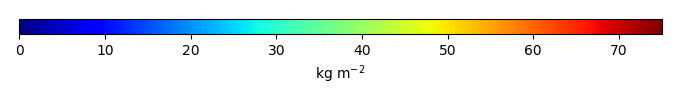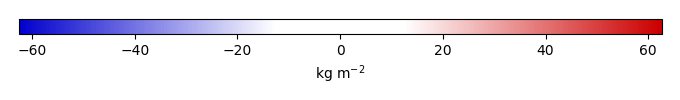# Mean State

Period Mean (original grids) [Pg]
Model Period Mean (intersection) [Pg]
Model Period Mean (complement) [Pg]
Benchmark Period Mean (intersection) [Pg]
Benchmark Period Mean (complement) [Pg]
Bias [kg m-2]
Bias Score 
Spatial Distribution Score 
Overall Score 
Benchmark [-] 76.5
CLM4 [-] 7.10 6.35 0.864 76.1 0.362 -24.5 0.405 0.154 0.279
CLM4.5 [-] 66.4 59.2 7.36 76.1 0.362 -5.19 0.590 0.607 0.599
CLM5 [-] 101. 90.3 10.0 76.1 0.362 6.17 0.533 0.297 0.415
Period Mean (original grids) [Pg]
Model Period Mean (intersection) [Pg]
Model Period Mean (complement) [Pg]
Benchmark Period Mean (intersection) [Pg]
Benchmark Period Mean (complement) [Pg]
Bias [kg m-2]
Bias Score 
Spatial Distribution Score 
Overall Score 
Benchmark [-] 113.
CLM4 [-] 62.4 38.4 22.5 113. 0.00185 -15.6 0.522 0.227 0.375
CLM4.5 [-] 111. 59.7 48.5 113. 0.00185 -11.2 0.574 0.673 0.624
CLM5 [-] 133. 70.5 59.6 113. 0.00185 -9.00 0.560 0.825 0.693
Period Mean (original grids) [Pg]
Model Period Mean (intersection) [Pg]
Model Period Mean (complement) [Pg]
Benchmark Period Mean (intersection) [Pg]
Benchmark Period Mean (complement) [Pg]
Bias [kg m-2]
Bias Score 
Spatial Distribution Score 
Overall Score 
Benchmark [-] 12.2
CLM4 [-] 67.8 3.17 66.2 12.2 -9.21 0.485 0.710 0.597
CLM4.5 [-] 96.4 4.96 93.3 12.2 -7.28 0.563 0.833 0.698
CLM5 [-] 91.8 5.31 88.9 12.2 -6.98 0.546 0.822 0.684
Period Mean (original grids) [Pg]
Model Period Mean (intersection) [Pg]
Model Period Mean (complement) [Pg]
Benchmark Period Mean (intersection) [Pg]
Benchmark Period Mean (complement) [Pg]
Bias [kg m-2]
Bias Score 
Spatial Distribution Score 
Overall Score 
Benchmark [-] 97.3
CLM4 [-] 4.45 4.30 0.467 97.2 0.0996 -29.4 0.386 0.0744 0.230
CLM4.5 [-] 66.3 60.0 7.26 97.2 0.0996 -11.7 0.590 0.776 0.683
CLM5 [-] 119. 110. 10.0 97.2 0.0996 4.55 0.545 0.395 0.470
Period Mean (original grids) [Pg]
Model Period Mean (intersection) [Pg]
Model Period Mean (complement) [Pg]
Benchmark Period Mean (intersection) [Pg]
Benchmark Period Mean (complement) [Pg]
Bias [kg m-2]
Bias Score 
Spatial Distribution Score 
Overall Score 
Benchmark [-] 5.12
CLM4 [-] 19.0 0.446 18.4 5.12 -17.3 0.401 0.0492 0.225
CLM4.5 [-] 47.1 2.27 44.3 5.12 -10.6 0.535 0.314 0.424
CLM5 [-] 44.2 3.46 40.1 5.12 -6.30 0.638 0.616 0.627
Period Mean (original grids) [Pg]
Model Period Mean (intersection) [Pg]
Model Period Mean (complement) [Pg]
Benchmark Period Mean (intersection) [Pg]
Benchmark Period Mean (complement) [Pg]
Bias [kg m-2]
Bias Score 
Spatial Distribution Score 
Overall Score 
Benchmark [-] 475.
CLM4 [-] 500. 79.0 421. 474. 1.51 -17.4 0.441 0.149 0.295
CLM4.5 [-] 984. 277. 706. 474. 1.51 -8.45 0.558 0.822 0.690
CLM5 [-] 1.06e+03 433. 622. 474. 1.51 -1.41 0.534 0.642 0.588
Period Mean (original grids) [Pg]
Model Period Mean (intersection) [Pg]
Model Period Mean (complement) [Pg]
Benchmark Period Mean (intersection) [Pg]
Benchmark Period Mean (complement) [Pg]
Bias [kg m-2]
Bias Score 
Spatial Distribution Score 
Overall Score 
Benchmark [-] 116.
CLM4 [-] 15.0 14.1 0.859 116. 0.373 -16.7 0.418 0.0745 0.246
CLM4.5 [-] 69.6 64.7 4.56 116. 0.373 -7.86 0.514 0.716 0.615
CLM5 [-] 123. 115. 7.60 116. 0.373 0.749 0.492 0.623 0.557
Period Mean (original grids) [Pg]
Model Period Mean (intersection) [Pg]
Model Period Mean (complement) [Pg]
Benchmark Period Mean (intersection) [Pg]
Benchmark Period Mean (complement) [Pg]
Bias [kg m-2]
Bias Score 
Spatial Distribution Score 
Overall Score 
Benchmark [-] 0.0131
CLM4 [-] 27.3 0.0156 26.9 0.0131 1.12 0.827 3.36e-22 0.414
CLM4.5 [-] 40.5 0.0331 39.8 0.0131 9.10 0.214 9.22e-23 0.107
CLM5 [-] 32.2 0.0178 31.5 0.0131 2.19 0.692 2.46e-23 0.346
Period Mean (original grids) [Pg]
Model Period Mean (intersection) [Pg]
Model Period Mean (complement) [Pg]
Benchmark Period Mean (intersection) [Pg]
Benchmark Period Mean (complement) [Pg]
Bias [kg m-2]
Bias Score 
Spatial Distribution Score 
Overall Score 
Benchmark [-] 46.1
CLM4 [-] 29.3 12.0 17.4 46.1 0.00725 -16.5 0.475 0.0997 0.287
CLM4.5 [-] 58.2 24.9 33.8 46.1 0.00725 -10.3 0.541 0.260 0.400
CLM5 [-] 75.2 36.0 39.5 46.1 0.00725 -4.90 0.560 0.579 0.570
Period Mean (original grids) [Pg]
Model Period Mean (intersection) [Pg]
Model Period Mean (complement) [Pg]
Benchmark Period Mean (intersection) [Pg]
Benchmark Period Mean (complement) [Pg]
Bias [kg m-2]
Bias Score 
Spatial Distribution Score 
Overall Score 
Benchmark [-] 0.232
CLM4 [-] 10.4 0.246 10.5 0.232 -0.0563 0.522 0.365 0.443
CLM4.5 [-] 13.2 0.260 13.6 0.232 0.211 0.517 0.628 0.572
CLM5 [-] 11.5 0.289 11.9 0.232 0.143 0.533 0.808 0.671

# Temporally integrated period mean

BENCHMARK MEANMODEL MEANBIASBIAS SCORESPATIAL TAYLOR DIAGRAMMODEL COLORS# Spatially integrated regional mean

MODEL COLORS# All Models

BenchmarkCLM4CLM4.5CLM5# Data Information

Approach: I just read the data from the original data file and converted the latitude arrange from original 90N->90S to 90S->90N. The unit was convert from original 0.1 KgC/m2 to KgC/m2

Temporal resolution: yearly

General information: This product was derived from Northern Circumpolar Soil Carbon Database V2.2 (NCSCDv22) in top 1m.

Spatial resolution: 0.5x0.5 degree